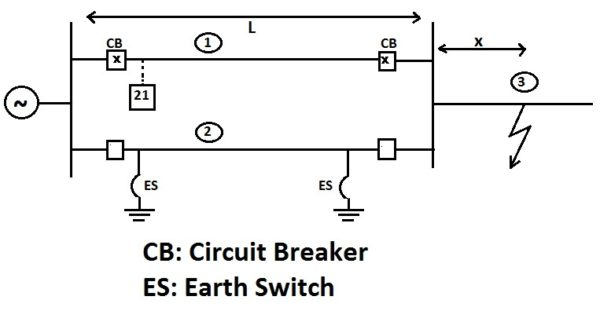# Over Reach of Distance Relay

## What is Meant by Over Reach?

To understand the meaning of over reach, let us take an example of double circuit line in which one line is out of service and earthed at both end by Earth Switch. In the figure below, the distance relay is supposed to protect the line 1 due to its impedance setting. Now suppose a fault take place in line 3 at a distance x as shown. In this case distance relay 21 is not expected to trip but if proper care is not taken then 21 may operate. This is called Over Reach. Mind the word over reach, the word itself means that the relay has actuated beyond its reach setting.“Thus a distance relay is said to over reach if the impedance measured by the relay is less than the apparent fault impedance.” Over reach is often expressed in percentage as below.

% Over Reach = (ZF – ZR)x100 / ZR

ZF = Effective Reach

ZR = Impedance Setting of Relay

In above example, effective reach of relay = (L+x). Effective reach means the length of line up to which the impedance measured by relay is under impedance setting.

Here, percentage over reach is (x/L)x100 %. Mind that we used length of line and distance of point of fault as impedance of line is proportional to length.

## Cause of Over Reach

This phenomena is mostly observed in double circuit line. In double circuit line, when one of the line is taken out of service and earthed ad both ends, then due to mutual coupling between the in-service line and out of service line, the impedance measurement of distance relay of in-service line do not remain accurate.

In such case, the effective reach of relay is given as

ZF = ZR+ Z0M / [ZL0(2ZL1+ZL0)]

ZR = Impedance setting of Relay

Z0M = Zero Sequence Impedance due to mutual coupling

ZL0 = Zero Sequence Impedance of protected line

ZL1 = Positive Sequence Impedance of protected line

Hence, percentage overreach = Z0Mx100 / [ZL0(2ZL1+ZL0)] %

For a typical 400 kV line, Z0M = 0.4, ZL1 = 0.46 and ZL0 = 0.76 Ohm/km.

Hence percentage over reach for 400 kV double circuit line when one line out and earthed = 16.4 %

Thus whenever one line of double circuit line is taken out and earthed for maintenance or other activity, distance relay setting is reduced by this over reach percentage i.e. 16.4% by some authorities. In other words, distance relay setting is reduced to 85.6 %. After normalization of line, the setting is again changed to 100 %. This practice is usually not adopted in India due to rare chance of fault in the first section of other line 3.

### One thought on “Over Reach of Distance Relay”

1.Abdul Azees.A says:

It is very helpful and effective

This site uses Akismet to reduce spam. Learn how your comment data is processed.